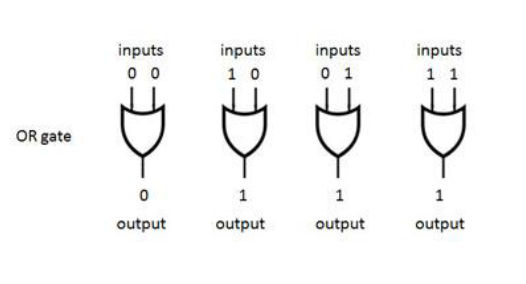# What Is an ALU and How an ALU Works | Arithmetic Logic Unit (ALU) - CodeTextPro

WORKING PRINCIPLE OF ALU

What is ALU (Arithmetic Logic Unit), Arithmetic Logic Unit (ALU): Definition, Design & Function, Arithmetic Logic Unit (ALU).

An arithmetic logic unit (ALU) represents the fundamental building block of the central processing unit of a computer. An ALU is a digital circuit used to perform arithmetic and logic operations.

What Is an ALU?
An arithmetic logic unit (ALU) is a digital circuit used to perform arithmetic and logic operations. It represents the fundamental building block of the central processing unit (CPU) of a computer. Modern CPUs contain very powerful and complex ALUs. In addition to ALUs, modern CPUs contain a control unit (CU).

Most of the operations of a CPU are performed by one or more ALUs, which load data from input registers. A register is a small amount of storage available as part of a CPU. The control unit tells the ALU what operation to perform on that data, and the ALU stores the result in an output register. The control unit moves the data between these registers, the ALU, and memory.

How an ALU Works:
An ALU performs basic arithmetic and logic operations. Examples of arithmetic operations are addition, subtraction, multiplication, and division. Examples of logic operations are comparisons of values such as NOT, AND, and OR.

All information in a computer is stored and manipulated in the form of binary numbers, i.e. 0 and 1. Transistor switches are used to manipulate binary numbers since there are only two possible states of a switch: open or closed. An open transistor, through which there is no current, represents a 0. A closed transistor, through which there is a current, represents a 1.

Operations can be accomplished by connecting multiple transistors. One transistor can be used to control a second one - in effect, turning the transistor switch on or off depending on the state of the second transistor. This is referred to as a gate because the arrangement can be used to allow or stop a current.

The simplest type of operation is a NOT gate. This uses only a single transistor. It uses a single input and produces a single output, which is always the opposite of the input. This figure shows the logic of the NOT gate:

Other gates consist of multiple transistors and use two inputs. The OR gate results in a 1 if either the first or the second input is a 1. The OR gate only results in a 0 if both inputs are 0. This figure shows the logic of the OR gate:How an OR gate processes binary data

The AND gate results in a 1 only if both the first and second input are 1s. This figure shows the logic of the AND gate:

The XOR gate, also pronounced X-OR gate, results in a 0 if both the inputs are 0 or if both are 1. Otherwise, the result is a 1. This figure shows the logic of the XOR gate: